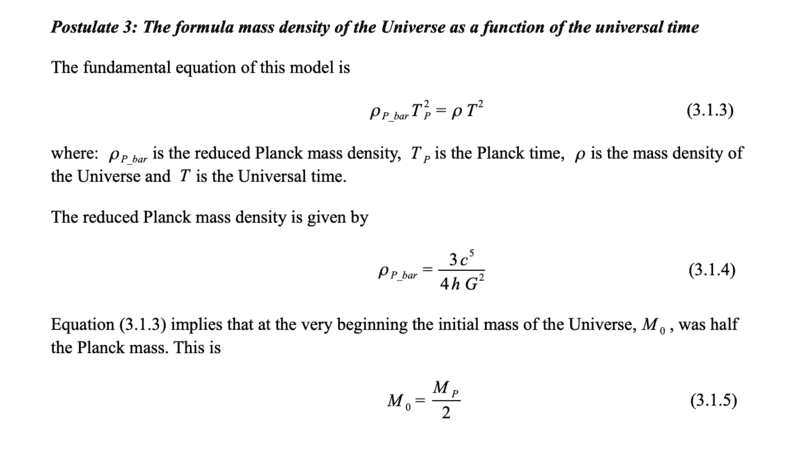# The Mystery of Equation (3.1.3) and the Origin of the Universe

• bobsan

#### bobsan

Homework Statement
How can we know that the initial mass of the universe was half of the Planck mass from the formula mass density of the Universe as a function of universal time?
Relevant Equations
(ρ reduced)(Tp)^2 = ρT^2
ρ reduced = 3c^5/(4hG^2)I tried setting the Universal time T = Tp as when T = 0 there was no universe and thought Tp would be the first instance of the universe, but I still can't figure out how equation (3.1.3) implies that M0 = Mp/2

Last edited by a moderator:
Homework Statement:: How can we know that the initial mass of the universe was half of the Planck mass from the formula mass density of the Universe as a function of universal time?
Relevant Equations:: (ρ reduced)(Tp)^2 = ρT^2
ρ reduced = 3c^5/(4hG^2)

View attachment 301814
I tried setting the Universal time T = Tp as when T = 0 there was no universe and thought Tp would be the first instance of the universe, but I still can't figure out how equation (3.1.3) implies that M0 = Mp/2
Hi @bobsan. Welcome to PF.

The reasoning in the attachment isn’t clear (well, it's missing actually). Not a familiar area for me but you could try this...

Consider the initial volume to be a sphere with diameter (or possibly radius) equal to the Planck length. Express this volume in terms of ℏ, G and c.

Multiply this by the density as given in equation 3.1.4. This gives you an expression for the initial mass. Compare this expression to the standard formula (expressed in in terms of ℏ, G and c)for the Planck mass.

If you’re lucky, you may find your expression for mass is half the Planck mass. No idea if that’s what’s intended though.

•bobsan
Where are you getting this from?

•vanhees71